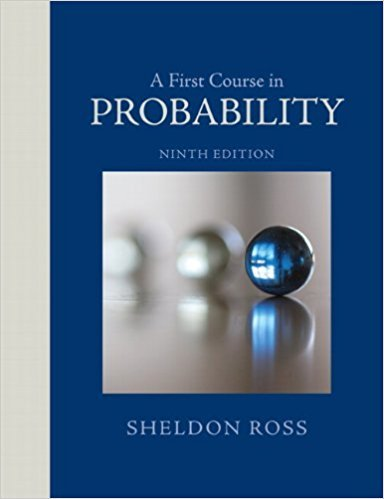×
Log in to StudySoup
Get Full Access to A First Course In Probability - 9 Edition - Chapter 4 - Problem 1p
Join StudySoup for FREE
Get Full Access to A First Course In Probability - 9 Edition - Chapter 4 - Problem 1p

Already have an account? Login here
×
Reset your password

# Two balls are chosen randomly from an urn containing 8ISBN: 9780321794772 63

## Solution for problem 1P Chapter 4

A First Course in Probability | 9th Edition

• Textbook Solutions
• 2901 Step-by-step solutions solved by professors and subject experts
• Get 24/7 help from StudySoup virtual teaching assistantsA First Course in Probability | 9th Edition

4 5 1 303 Reviews
19
0
Problem 1P

Problem 1P

Two balls are chosen randomly from an urn containing 8 white, 4 black, and 2 orange balls. Suppose that we win \$2 for each black ball selected and wc lose \$1 for each white ball selected. Let A denote our winnings. What are the possible values of X, and what are the probabilities associated with each value?

Step-by-Step Solution:

Solution:

Step 1 of 2:

Two balls are selected randomly from an urn contains 8 white, 4 black, and 2 orange balls.

We win \$2 for each black ball selected and we lose \$1 for each white ball selected.

We have find the possible values of X and their corresponding probabilities.

Step 2 of 2

##### ISBN: 9780321794772

Unlock Textbook Solution

Enter your email below to unlock your verified solution to:

Two balls are chosen randomly from an urn containing 8